## Spammy advertising best reason for switching to HTTPS

While transiting at Schiphol and using the airport wifi, I noticed the sudden appearance of a bunch of adverts on normally advert-free websites. For example:Some investigation indicated that this time the adverts were not injected via Google Analytics, but instead attached directly into the HTML content of the page. First at the top we have some injected CSS: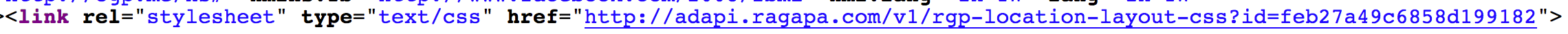Then at the bottom we have the real payload, injected JavaScript code: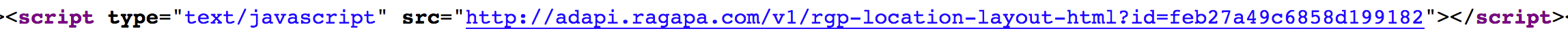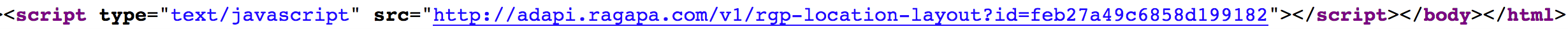It appears this is the same type of advertising afflicting AT&T hotspots – information gleaned from Jonathan Meyer, whose website describing the issue is itself also affected by the Schipol adverts:Again it seems that given the large scale involved, someone, somewhere – perhaps including a company called “RaGaPa” who seem to be responsible for the ads – is making quite a bit of money through unsavory and perhaps legally questionable means.

Just in case the adverts on their own are not spammy enough, the icon at the top right of the adverts link to the following explanation, casually noting that in addition to standard user tracking and user history ad serving, “You may also be redirected to sponsor’s websites or welcome pages at a set frequency”:Perhaps the real take-home though is that HTTPS sites are, again, not affected by this: content injection of this type is not possible on sites served using HTTPS without defeating the certificate authority chain or sidestepping it with other kinds of trickery. Digital Sinology recently moved to HTTPS, so is not affected by this particular attack.

## Classical Chinese Digital Humanities

By Donald Sturgeon

### List of tutorials# Classical Chinese DH: Python programming and ctext.org API¶

By Donald Sturgeon

# Variables¶

Variables are named entities that contain some kind of data that can be changed at a later date. You can choose (almost) any name for a variable as long as it is not the same as a reserved word (i.e. has some special meaning in the Python language), though typically these names will be composed of letters a-z. The names given to variables have no special meaning to the computer, but giving variables names that describe their function in a particular program is usually very helpful to the programmer – and to anyone else who may look at your code. Spaces cannot be part of a variable name, so sometimes other allowed characters (e.g. “_”) are used instead for clarity. Although it is possible to use non-English characters for variable names, this is generally inadvisable as it may cause compatibility problems when running the same program on another computer.

A value is assigned to a variable using the syntax “variable_name = new_value“.

In :
```number_of_people = 5
print(number_of_people)
```
```5
```

A variable only ever has one value at a time. When we assign a second value to a variable, anything that was in it before is lost.

In :
```number_of_people = 5
number_of_people = 15
print(number_of_people)
```
```15
```

In Python, variable names are case sensitive, so as far as Python is concerned, a variable called “thisone” is completely different from a variable called “ThisOne” or “THISONE”.

In :
```test = 1
print(Test)  # This will not work and will give an error, because "test" and "Test" are different variables
```
```---------------------------------------------------------------------------
NameError                                 Traceback (most recent call last)
<ipython-input-3-c8ddc105ba6a> in <module>()
1 test = 1
----> 2 print(Test)  # This will not work and will give an error, because "test" and "Test" are different variables

NameError: name 'Test' is not defined```
In :
```thisone = 5
ThisOne = 10
print(thisone)
print(ThisOne)
```
```5
10
```

We can perform basic arithmetic on variables and numbers using symbols representing arithmetic operators:

 + Add – Subtract / Divide * Multiply
In :
```number_of_people = 5
pages_per_person = 12
print(number_of_people * pages_per_person)
```
```60
```

# Strings¶

One of the most important units of text in most programming languages is the string: an ordered sequence of zero or more characters. Strings can be “literal” strings – string data typed in to a program – or the contents of a variable.

Literal strings have to be enclosed in special characters so that Python knows exactly which part of what appears in the program belongs to the string being defined. You can use either a pair of double quotation marks (“…”) or single quotation marks (‘…’) for this. (Note: most programming languages including Python will not allow the use of ‘full-width’ Chinese / CJK punctuation characters for this purpose.)

In :
```print("學而時習之")
```
```學而時習之
```
In :
```analects_1_1 = "子曰：「學而時習之，不亦說乎？有朋自遠方來，不亦樂乎？人不知而不慍，不亦君子乎？」"
print(analects_1_1)
```
```子曰：「學而時習之，不亦說乎？有朋自遠方來，不亦樂乎？人不知而不慍，不亦君子乎？」
```

Two strings can be joined together (concatenated) using the “+” operator to give a new string:

In :
```analects_1_3 = "子曰：「巧言令色，鮮矣仁！」"
print(analects_1_1 + analects_1_3)
```
```子曰：「學而時習之，不亦說乎？有朋自遠方來，不亦樂乎？人不知而不慍，不亦君子乎？」子曰：「巧言令色，鮮矣仁！」
```

In Python, each variable has a particular “type”. The most common types are “string”, “integer” (…,-2,-1,0,1,2,…), and “float” (any real number, e.g. 3.1415, -26, …). When joining a string and a number using “+”, we need to specify that the number should be changed into a string:

In :
```print(analects_1_1 + 5) # This will not work
```
```---------------------------------------------------------------------------
TypeError                                 Traceback (most recent call last)
<ipython-input-9-0eb38b4432f4> in <module>()
----> 1 print(analects_1_1 + 5) # This will not work

TypeError: Can't convert 'int' object to str implicitly```
In :
```print(analects_1_1 + str(5))
```
```子曰：「學而時習之，不亦說乎？有朋自遠方來，不亦樂乎？人不知而不慍，不亦君子乎？」5
```

Sometimes we may need to include “special” characters in our strings, like the return character (\n), or tab character (\t). Also, if we need to include quotation marks as part of our string, we can do this by putting a backslash before each quotation mark (\”).

In :
```output_string = "第一行\n第二行\n\"第三行\""
print(output_string)
```
```第一行

"第三行"
```

## Doing things with strings¶

Once we have data in a string, we can manipulate it in various ways. Often a program will be designed to work on arbitrary string input, i.e. the program will not know in advance what strings it will be asked to work with. So we need ways of finding out basic things about the string. First of all, how long (in characters) is the string:

In :
```print(len(analects_1_1))
```
```41
```

N.B. “Characters” here means characters in the technical string sense. It includes, for example, all punctuation symbols, and other “special characters” that may be in the string (such as characters representing line breaks).

We can take a single character from a string and create a new string containing just that character using the notation “string_variable[m]“, where m is a number describing the position of the character we want to copy from the string.

N.B. In Python (and many other languages), the characters in a string are numbered starting from 0. So if a string has a length of 5, its characters are numbered 0, 1, 2, 3, and 4.

In :
```print(analects_1_1)
```
```子
```
In :
```print(analects_1_1)
```
```而
```

If we use a negative value for m, we can do the same thing but counting backwards from the end of the string:

In :
```print(analects_1_1[-3])
```
```乎
```

Another useful basic function is making a new string from some part of an existing string – this is called a “substring”.
In Python, we get a substring of a string starting at position m and ending just before position n using the notation “string_variable[m:n]”:

In :
```print(analects_1_1[0:1])
```
```子
```
In :
```print(analects_1_1[1:2])
```
```曰
```
In :
```print(analects_1_1[0:2])
```
```子曰
```
In :
```print(analects_1_1[4:15])
```
```學而時習之，不亦說乎？
```
In :
```print(len(analects_1_1[4:15]))
```
```11
```

If we want to count characters from the end of a string, instead of from the beginning, we can use a negative number for m (meaning “start from –m characters before the end of the string”) and either omit n entirely (meaning “up to the end of the string”) or use a negative number for n (meaning “up to –n characters before the end of the string”):

In :
```print(analects_1_1[-7:])
```
```不亦君子乎？」
```
In :
```print(analects_1_1[-7:-1])
```
```不亦君子乎？
```

There are many other functions for doing things with strings – we will see more of these in week 3. In the meantime, two useful functions are count(), which returns the number of times one string occurs within another string.

In :
```input_text = "道可道，非常道。"
print(input_text.count("道"))
```
```3
```
In :
```print(input_text.count("道可"))
```
```1
```

Another is replace(), which creates a new string in which all matching occurrence of a substring have been replaced by something else. The general form looks something like this:

string_to_search_in.replace(thing_to_search_for, thing_to_replace_with)

N.B. This function does not change the data in the original variable. It just returns new data with the substitution made.

In :
```input_text = "道可道，非常道。"
print(input_text.replace("道", "名"))
```
```名可名，非常名。
```
In :
```print(input_text)  # Note: the input_text variable still contains the same data
```
```道可道，非常道。
```

## Lists¶

Lists are another kind of variable that work a lot like strings, except that whereas each location within a string is always exactly one character, each location in a list can be any kind of value, such as a number or a string.

We can make a list variable by separating each list element with commas and enclosing the whole lot in square brackets.

In :
```days_of_week = ["星期天", "星期一", "星期二", "星期三", "星期四", "星期五", "星期六"]
print(days_of_week)
```
```['星期天', '星期一', '星期二', '星期三', '星期四', '星期五', '星期六']
```

In Python, the items stored in a list are numbered starting from 0. This means if we have 7 items in our list, they are numbered 0, 1, 2, 3, 4, 5, and 6.

In :
```print(days_of_week)
```
```星期天
```
In :
```print(days_of_week)
```
```星期六
```

If we try to use an item that isn’t in our list, we will get an error.

In :
```print(days_of_week)
```
```---------------------------------------------------------------------------
IndexError                                Traceback (most recent call last)
<ipython-input-30-e6a905455149> in <module>()
----> 1 print(days_of_week)

IndexError: list index out of range```

We can set the value of items in a list just like regular variables.

In :
```days_of_week = "禮拜三"
print(days_of_week)
```
```['星期天', '星期一', '星期二', '禮拜三', '星期四', '星期五', '星期六']
```

Often when we process lists in a program, we will need to find out how long the list is. This is because our programs will usually be designed to work with any input of a certain type (for example, to work with any text, not just one we’ve chosen in advance), and so we will only find out many items there are in a particular list when our program is actually run. The len() function tells us how many items are in our list.

In :
```print(len(days_of_week))
```
```7
```

Remember that when we use len() on a string, it will give us the length of the string in numbers of characters. So for our days_of_week example:

In :
```print(len(days_of_week))
print(len(days_of_week))
# etc.
```
```3
3
```

Make sure you understand why we get this answer here.

## True and false¶

Boolean logic – i.e. logic in which things are either true or false – is central to most commonly used programming languages. Typically programs make decisions as to what to do next based on whether some particular expression (e.g. comparison of variables) is true or false. Some basic comparison operators are:

 == equals > greater than < less than >= greater than or equal to <= less than or equal to

N.B. Assignment, e.g. a=1, uses a single “=”, whereas comparison, e.g. a==2, uses a double “==”.

In :
```print(5>2)
```
```True
```
In :
```print(5>7)
```
```False
```
In :
```number_of_pages = 5*12
print(number_of_pages == 60)
```
```True
```
In :
```print(analects_1_1)
print(analects_1_3)
print(analects_1_1[0:2] == analects_1_3[0:2])   # If you're not sure why, try using print() on the two sides of the equation
```
```子曰：「學而時習之，不亦說乎？有朋自遠方來，不亦樂乎？人不知而不慍，不亦君子乎？」

True
```
In :
```print(analects_1_1[0:7] == analects_1_3[0:7])   # If you're not sure why, try using print() on the two sides of the equation
```
```False
```

Often we want to know whether something occurs anywhere within a string. One way to do this is using the in operator. (We will look at more sophisticated types of searching for more complex patterns next week.)

In :
```text_to_search_in = "有子曰：「其為人也孝弟，而好犯上者，鮮矣；不好犯上，而好作亂者，未之有也。君子務本，本立而道生。孝弟也者，其為仁之本與！」"
print("孝弟" in text_to_search_in)
```
```True
```
In :
```print("仁義" in text_to_search_in)
```
```False
```

## Making decisions¶

Now that we can compare things, we can start to change what we do depending on the outcome of these comparisons.

The simplest type of decision is an “if … then …” decision: if something is true, then do something (otherwise don’t do it).

In :
```if(3>2):
print("3 is greater than 2")

if(2>3):
print("2 is greater than 3")
```
```3 is greater than 2
```

In Python, indentation (one or more spaces from the left-hand margin) is used to mark blocks of code (sequences of instructions) which are to be followed together. For example:

In :
```if(3>2):
print("3 is greater than 2")
print("This is also executed if 3>2")

if(2>3):
print("2 is greater than 3")

print("This is *always* executed, because it is outside of both 'if' blocks")
```
```3 is greater than 2
This is also executed if 3>2
This is *always* executed, because it is outside of both 'if' blocks
```

The “else” keyword can be used after an “if” to do one thing if a condition is true, and some other thing if it is not true:

In :
```text1 = "Some text"
text2 = "Some other text"
if(len(text1) > len(text2)):
print("text 1 is longer than text 2")
else:
print("text 1 is not longer than text 2")  # It might be exactly the same length though - try changing the text in text1 and text2
```
```text 1 is not longer than text 2
```

Also useful are logical operators “and“, “or“, and “not“. These allow us to make more complex decisions based on several factors.

Python expression Result
A and B True if A is True and B is also True – otherwise False
A or B True if A is True or B is True, or both are True – otherwise False
not A True if A is False – otherwise False

N.B. Matching pairs of brackets are used in complex expressions to remove ambiguity: the innermost brackets are always evaluated first. For instance:

``````(a and b) or c   # This will only be true when either: 1) a and b are both true; or 2) c is true
a and (b or c)   # This will only be true when *both*: 1) a is true; and 2) either b is true or c is true
a and b or c     # ???? Don't write this - it's not obvious which of the previous two lines it corresponds to

``````

Suggestion: don’t write things like “a and b or c” without brackets, as these are confusing. (There are rules that mean they are not ambiguous, but instead of worrying about these now, always use brackets when mixing and, or, and not in an expression.)

Experiment with changing the values of the three variables below, making sure you understand why you get different results.

In :
```is_raining = False
will_rain_later = True
i_am_going_out = True

print(is_raining or will_rain_later)
print((is_raining or will_rain_later) and i_am_going_out)

if ((not is_raining) and will_rain_later) and i_am_going_out:
print("Did you see the forecast?")

if (is_raining or will_rain_later) and i_am_going_out:
print("Better take an umbrella!")
```
```True
True
Did you see the forecast?
Better take an umbrella!
```

## Repeating instructions¶

A lot of the power of digital methods comes from the fact that once we have a program that performs some task on an arbitrary object of some kind, we can easily have the computer perform that same task on large numbers of objects. One of the simplest ways of repeating instructions is the “for” loop. Just like with if, in Python we use indentation to indicate exactly which instructions we want repeated. For loops are often used with the range() function:

In :
```for some_variable in range(0,5):
print(some_variable)
```
```0
1
2
3
4
```

The range() function takes two parameters that determine what range of numbers we want: this first parameter determines the number we want to begin with, and the second determines the end of the range. Note: the “end” parameter to range() is not inclusive – so range(0,3) will give us a range with the numbers 0,1,2 but not 3.

As well as range(), we can also loop over each character in a string, or over each item in a list (i.e. do some processing for each character or item).

In :
```for day in days_of_week:
print(day)
```
```星期天

```

Working with characters in a string is just as easy – we can use the format “for character_variable in string:”

In :
```my_string = "道可道，非常道。"
for my_character in my_string:
print(my_character)
```
```道

，

。
```

However, sometimes we also need to know where (i.e. at what index) we are in a string.

In :
```for character_index in range(0,len(my_string)):
print("Index " + str(character_index) + " in our string is: " + my_string[character_index])
```
```Index 0 in our string is: 道
Index 1 in our string is: 可
Index 2 in our string is: 道
Index 3 in our string is: ，
Index 4 in our string is: 非
Index 5 in our string is: 常
Index 6 in our string is: 道
Index 7 in our string is: 。
```

Sometimes we will need to have one loop inside another loop. In this case, we use progressively larger indentations to indicate which instructions should be repeated in which loop.

In :
```for x in range(1,4):
print("Starting sums with x = " + str(x))
for y in range(10,14):
z = x * y
print(str(x) + "*" + str(y) + "=" + str(z))
print("Finished sums with x = " + str(x))
print("Finished all the sums")
```
```Starting sums with x = 1
1*10=10
1*11=11
1*12=12
1*13=13
Finished sums with x = 1
Starting sums with x = 2
2*10=20
2*11=22
2*12=24
2*13=26
Finished sums with x = 2
Starting sums with x = 3
3*10=30
3*11=33
3*12=36
3*13=39
Finished sums with x = 3
Finished all the sums
```

## Getting Chinese texts¶

If you already have a copy of a text you’d like to process, you can easily read it from a text file into a string variable. However, as the formatting of each text may be different, the exact steps needed to process the text may differ slightly in each case. We will look at how to deal with this in detail next time when we look at regular expressions, since these provide powerful tools to quickly reorganize textual data.

An alternative way of getting textual data for historical texts is to use the ctext.org API, which lets us get the text for many historical texts in a consistent format. Texts are identified using a URN (Uniform Resource Name) – you can see this written at the bottom of the page when you view the corresponding text on ctext.org.

To make it easier to access these texts, we can install a specialized Python module to access the API. Before we can use it, we have to install it. Python makes this very easy to do, and it only needs to be done once; if you followed the instructions in the first tutorial, you should already have this module installed.

Once installed, we can use functions from this module to read textual data into Python variables. If we don’t care about the structure of a text, but only its contents, we can read the text into a single list of strings, each containing a single paragraph, like this:

In :
```from ctext import *  # This lets us get Chinese texts from http://ctext.org
setapikey("demo")    # This allows us access to the data used in these tutorials

passages = gettextasparagrapharray("ctp:analects")

print("Total number of passages: " + str(len(passages)))
print("First passage is: " + passages)
print("Second passage is: " + passages)
print("Last passage is: " + passages[-1])
```
```Total number of passages: 503
First passage is: 子曰：「學而時習之，不亦說乎？有朋自遠方來，不亦樂乎？人不知而不慍，不亦君子乎？」
Second passage is: 有子曰：「其為人也孝弟，而好犯上者，鮮矣；不好犯上，而好作亂者，未之有也。君子務本，本立而道生。孝弟也者，其為仁之本與！」
Last passage is: 子曰：「不知命，無以為君子也。不知禮，無以立也。不知言，無以知人也。」
```

To read or write to a file, we must first open it. When we open a file, we must specify both the name of the file, and whether we want to read data from it (“r”), or write data to it (“w”). The file.write() function works very much like print(), but writes its output directly to the file instead of to the screen. When writing to a file, however, we need to explicitly include return characters at the end of each line using “\n”. The file.read() function reads all the data from the file, which you can assign to a python variable.

N.B. Be careful when opening files! When you use “w” to open a file for writing, any file with that name in the same folder as your Python Notebook will immediately be replaced with a new, empty file.

In :
```file = open("week2_testfile.txt", "w", encoding="utf-8") # N.B. "w" here means we will open this file and write to it. If the file exists, it will immediately be deleted.
file.write("第一行\n第二行")
file.close()
```

Now take a look in Windows Explorer or Mac OS Finder and make sure you can see where this file is on your computer. It will be helpful for you to know in which folder Python expects files to be by default.

In :
```file = open("week2_testfile.txt", "r", encoding="utf-8") # "r" means we will open this file for reading, and won't be able to modify it
file.close()
print(data_from_file)
```
```第一行

```

## Exercises¶

1.i) Write a program using a for loop to output all of the substrings of length 2 contained in the variable input_string. Your program should produce output like this:

``````天命

，率

...
...

In :
```input_string = "天命之謂性，率性之謂道，修道之謂教。道也者，不可須臾離也，可離非道也。"

```

1.ii) Now modify your program so that you first define a variable called “substring_length” containing a number determining the length of substring to be listed. Your new program should still give the same output when run with “substring_length=2”, but should also work with substring_length set to 3, 4, etc. Remember, every line that your program outputs should have exactly substring_length characters in it (including punctuation characters).

1.iii) Modify your program again so that on each line it prints the total number of times that the substring occurs in input_string. For instance, each line beginning “之謂” should now read “之謂 3”, since “之謂” occurs three times in this string.

1.iv) Run your program again, but now use this slightly longer text instead:

``````input_string = "天命之謂性，率性之謂道，修道之謂教。道也者，不可須臾離也，可離非道也。是故君子戒慎乎其所不睹，恐懼乎其所不聞。莫見乎隱，莫顯乎微。故君子慎其獨也。喜怒哀樂之未發，謂之中；發而皆中節，謂之和；中也者，天下之大本也；和也者，天下之達道也。致中和，天地位焉，萬物育焉。"

``````

Look at what the most frequent 2-grams are with this text. As before, some of them will include punctuation. Do any of these frequent 2-grams which include punctuation relate to facts about the language?

2.i) In the cell below, write a program to find and print out all passages in the Analects that include the term “仁”.

Note: if you’ve run the example program under Getting Chinese texts, the data is already stored in the passages variable.

In :
```# Your code goes here!
```

2.ii) Modify your program so it instead lists only passages that mention both terms “仁” and “義”.

2.iii) Modify your program so it instead lists only passages that mention either the term “愛人” or “知人” but not both.

3) Write another program (if you like, you can copy and paste your answer to the previous question and modify it) to determine which passage in the Analects mentions the term “禮” the greatest number of times.

Hint: Use one variable to track the greatest number of times “禮” has appeared, and another to track which passage it appeared in.

In :
```# Your code goes here!
```

4.i) Write a program in the cell below to store the full text of the Analects into a file on your computer called “analects.txt”. Put each paragraph on its own line, and in front of each paragraph put firstly the number of the paragraph, starting at 1, and secondly the length of the paragraph in characters. Separate each of these three pieces of data with a tab character. The beginning of your file should look like this:

```1   41   子曰：「學而時習之，不亦說乎？有朋自遠方來，不亦樂乎？人不知而不慍，不亦君子乎？」
2   61   有子曰：「其為人也孝弟，而好犯上者，鮮矣；不好犯上，而好作亂者，未之有也。君子務本，本立而道生。孝弟也者，其為仁之本與！」
```
In :
```# Your code goes here!
```
• Open the file in a text editor (e.g. Notepad for Windows, TextEdit for Mac OS X – usually double-clicking on the file you’ve created will do this) and check that the output looks correct.

4.ii) Modify your program so that the character counts only include Chinese characters, i.e. do not count punctuation characters.

4.iii) (Optional) If you have Excel or another spreadsheet program on your computer, try importing the file into it so that you get separate columns for the paragraph number, length, and content. (This may or may not be easy depending on your operating system and spreadsheet program. If you encounter encoding issues, try copying all of the data from the text editor straight into a blank spreadsheet instead.)

In :
```# Your code goes here!
```

5) [Harder] Which passage in the Analects contains a character repeated more frequently than any other character in any other passage – and what is the character?
It will help if you firstly think carefully about what you need to keep track of between passages in order to answer this question.

In :
```# Your code goes here!
```

Bonus question

This section is optional as it includes several things we haven’t covered yet and will look at later on in when we look more closely at structured data.

The program below uses a dictionary variable to count all of the 1-grams in the Analects. [A dictionary variable is very similar to a list, except that its items are not numbered 0,1,2,… but instead indexed using arbitrary strings – for instance, my_dictionary[“論語”], which might contain a string value such as “analects”, or a number like 32. The term “dictionary” here is metaphorical, i.e. a dictionary variable often does not contain translations of words from one language to another – though this is one possible use case.]

It then uses the pandas library to select the top ten most frequent 1-grams, and the matplotlib library to draw a bar chart of this data.

Read through the code, and see if you can modify it to find the most frequent 2-grams, 3-grams, etc.

In :
```import numpy as np
import matplotlib.pyplot as plt

# The next line tells the matplotlib library to display its output in our Jupyter notebook
%matplotlib inline
from ctext import *
import pandas as pd
import matplotlib as mpl

# Unfortunately some software still has difficulty dealing with Chinese.
# Here we may need to tell matplotlib to use a specific font containing Chinese characters.
import platform
if platform.system() == 'Darwin':   # I.e. if we're running on Mac OS X
mpl.rcParams['font.family'] = "STFangsong"
else:
mpl.rcParams['font.family'] = "SimHei"

mpl.rcParams['font.size'] = 20

chapterdata = gettextasparagrapharray("ctp:analects")

# Use a dictionary variable to keep track the count of each character we see
character_count = {}

# For each paragraph of the chapter data that we downloaded, do the following:
for paragraphnumber in range(0, len(chapterdata)):
for char in range(0,len(chapterdata[paragraphnumber])):
this_character = chapterdata[paragraphnumber][char:char+1]
# Don't bother counting punctuation characters
if this_character not in ["，", "。", "：", "；", "「", "」", "？"]:
if this_character in character_count:
new_count = character_count[this_character] + 1
else:
new_count = 1
character_count[this_character] = new_count

s = pd.Series(character_count)
s.sort_values(0, 0, inplace=True)

s[:10].plot(kind='barh')
print(s[:10])
```
```子    973

dtype: int64
```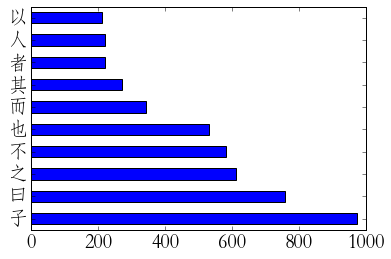## Classical Chinese DH: Getting Started

By Donald Sturgeon

This is the first in a series of online tutorials introducing basic digital humanities techniques using the Python programming language and the Chinese Text Project API. These tutorials are based in part on material covered in the course CHNSHIS 202: Digital Methods for Chinese Studies, which I teach at Harvard University’s Department of East Asian Languages and Civilizations.

Intended audience: People with some knowledge of Chinese literature and an interest in digital humanities; no programming experience necessary.

Format: Most of these tutorials will consist of a Jupyter Notebook file. These files contain a mixture of explanations and code that can be modified and run from within your web browser. This makes it very easy to modify, play with, and extend all of the example code. You can also read the tutorials online first (you’ll need to download the files in order to run the code and do the exercises though).

### Getting started

To use this series of tutorials, you need to first complete the following steps:

1. Install Python (programming language) and Jupyter (web browser based interface to Python). The recommended way to do this is by installing the Anaconda distribution, which will automatically install Python, Jupyter, and many other things we need. For these tutorials, you should install the Python 3.x version of Anaconda (not the 2.7 version).
2. Install the ctext module. To do this, after installing Anaconda, open Command Prompt (Windows) or Terminal (Mac OS X), and then type:
`pip install ctext [return]`
3. Create a folder to contain your Python projects. To follow a tutorial, first download the .ipynb Jupyter Notebook file and save it into this folder.
4. Start up the Jupyter environment. One way to do this is opening the Command Prompt (Windows) or Terminal (Mac OS X), and then typing:
`jupyter notebook [return]`
6. The Jupyter system works by having a server program which runs in the background (if you start Jupyter as described above, you can see it running in the Terminal / Command Prompt window), which is then accessed using a web browser. This means that when you close your web browser, Jupyter is still running until you stop the server process. You can stop the server process by opening the Terminal / Command Prompt window and pressing Control-C twice (i.e. holding down the “Control” key and pressing the C key twice).

Below is the Jupyter notebook for this tutorial. Since the first tutorial focuses on how to use the Jupyter environment, you should download and open this notebook in Jupyter rather than trying to follow it online.

# Classical Chinese DH: Getting started¶

By Donald Sturgeon

### Welcome to our first Jupyter Notebook!¶

A notebook is a hypertext document containing a mixture of textual content (like the part you’re reading now) and computer programs – lists of instructions written in a programming language (in our case, the Python language) – as well as the output of these programs.

### Using the Jupyter environment¶

Before getting started with Python itself, it’s important to get some basic familiarity with the user interface of the Jupyter environment. Jupyter is fairly intuitive to use, partly because it runs in a web browser and so works a lot like any web page. Basic principles:

• Each “notebook” displays as a single page. Notebooks are opened and saved using the menus and icons shown within the Jupyter window (i.e. the menus and icons under the Jupyter logo and icon, not the menus / icons belonging to your web browser).

• Notebooks are made up of “cells”. Each cell is displayed on the page in a long list, one below another. You can see which parts of the notebook belong to which cell by clicking once on the text – when you do this, this will select the cell containing the text, and show its outline with a grey line.

• Usually a cell contains either text (like this one – in Jupyter this is called a “Markdown” cell), or Python code (like the one below this one).

• You can click on a program cell to edit it, and double-click on a text cell to edit it. Try double-clicking on this cell.

• When you start editing a text cell, the way it is displayed changes so that you can see (and edit) any formatting codes in it. To return the cell back to the “normal” prettified display, you need to “Run” it. You can run a cell by either:

• choosing “Run” from the “Cell” menu above,
• pressing shift-return when the cell is selected, or
• clicking the “Run cell” icon.
• “Run” this cell so that it returns to the original mode of display.
In :
```for number in range(1,13):
print(str(number) + "*" + str(number) + " = " + str(number*number))
```
```1*1 = 1
2*2 = 4
3*3 = 9
4*4 = 16
5*5 = 25
6*6 = 36
7*7 = 49
8*8 = 64
9*9 = 81
10*10 = 100
11*11 = 121
12*12 = 144
```

The program in a cell doesn’t do anything until you ask Jupyter to run (a.k.a. “execute”) it – in other words, ask the system to start following the instructions in the program. You can execute a cell by clicking somewhere in it so it’s selected, then choosing “Run” from the “Cell” menu (or by pressing shift-return).

When you run a cell containing a Python program, any output that the program generates is displayed directly below that cell. If you modify the program, you’ll need to run it again before you will see the modified result.

A lot of the power of Python and Jupyter comes from the ability to easily make use of modules written by other people. Modules are included using lines like “from … import *”.
A module needs to be installed on your computer before you can use it; many of the most commonly used ones are installed as part of Anaconda.

“Comments” provide a way of explaining to human readers what parts of a program are supposed to do (but are completely ignored by Python itself). Typing the symbol # begins a comment, which continues until the end of the line.

N.B. You must install the “ctext” module before running the code below. If you get the error “ImportError: No module named ‘ctext'” when you try to run the code, refer to the instructions for how to install the ctext module.

In :
```from ctext import *  # This module gives us direct access to data from ctext.org
setapikey("demo")    # This allows us access to the data used in these tutorials

paragraphs = gettextasparagrapharray("ctp:analects/xue-er")

print("This chapter is made up of " + str(len(paragraphs)) + " paragraphs. These are:")

# For each paragraph of the chapter data that we downloaded, do the following:
for paragraphnumber in range(0, len(paragraphs)):
print(str(paragraphnumber+1) + ". " + paragraphs[paragraphnumber])
```
```This chapter is made up of 16 paragraphs. These are:
1. 子曰：「學而時習之，不亦說乎？有朋自遠方來，不亦樂乎？人不知而不慍，不亦君子乎？」
2. 有子曰：「其為人也孝弟，而好犯上者，鮮矣；不好犯上，而好作亂者，未之有也。君子務本，本立而道生。孝弟也者，其為仁之本與！」
3. 子曰：「巧言令色，鮮矣仁！」
4. 曾子曰：「吾日三省吾身：為人謀而不忠乎？與朋友交而不信乎？傳不習乎？」
5. 子曰：「道千乘之國：敬事而信，節用而愛人，使民以時。」
6. 子曰：「弟子入則孝，出則弟，謹而信，汎愛眾，而親仁。行有餘力，則以學文。」
7. 子夏曰：「賢賢易色，事父母能竭其力，事君能致其身，與朋友交言而有信。雖曰未學，吾必謂之學矣。」
8. 子曰：「君子不重則不威，學則不固。主忠信，無友不如己者，過則勿憚改。」
9. 曾子曰：「慎終追遠，民德歸厚矣。」
10. 子禽問於子貢曰：「夫子至於是邦也，必聞其政，求之與？抑與之與？」子貢曰：「夫子溫、良、恭、儉、讓以得之。夫子之求之也，其諸異乎人之求之與？」
11. 子曰：「父在，觀其志；父沒，觀其行；三年無改於父之道，可謂孝矣。」
12. 有子曰：「禮之用，和為貴。先王之道斯為美，小大由之。有所不行，知和而和，不以禮節之，亦不可行也。」
13. 有子曰：「信近於義，言可復也；恭近於禮，遠恥辱也；因不失其親，亦可宗也。」
14. 子曰：「君子食無求飽，居無求安，敏於事而慎於言，就有道而正焉，可謂好學也已。」
15. 子貢曰：「貧而無諂，富而無驕，何如？」子曰：「可也。未若貧而樂，富而好禮者也。」子貢曰：「《詩》云：『如切如磋，如琢如磨。』其斯之謂與？」子曰：「賜也，始可與言詩已矣！告諸往而知來者。」
16. 子曰：「不患人之不己知，患不知人也。」
```

‘Variables’ are named entities that contain some kind of data that can be changed at a later date. We will look at these in much more detail over the next few weeks. For now, you can think of them as named boxes which can contain any kind of data.

Once we have data stored in a variable (like the ‘paragraphs’ variable above), we can start processing it in whatever way we want. Often we use other variables to track our progress, like the ‘longest_paragraph’ and ‘longest_length’ variables in the program below.

In :
```longest_paragraph = None # We use this variable to record which of the paragraphs we've looked at is longest
longest_length = 0       # We use this one to record how long the longest paragraph we've found so far is

for paragraph_number in range(0, len(paragraphs)):
paragraph_text = paragraphs[paragraph_number];
if len(paragraph_text)>longest_length:
longest_paragraph = paragraph_number
longest_length = len(paragraph_text)

print("The longest paragraph is paragraph number " + str(longest_paragraph+1) + ", which is " + str(longest_length) + " characters long.")
```
```The longest paragraph is paragraph number 15, which is 93 characters long.
```

Modules allow us to do powerful things like Principle Component Analysis (PCA) and machine learning without having to write any code to perform any of the complex mathematics which lies behind these techniques. They also let us easily plot numerical results within the Jupyter notebook environment.

For example, the following code (which we will go through in much more detail in a future tutorial – don’t worry about the contents of it yet) plots the frequencies of the two characters “矣” and “也” in chapters of the Analects versus chapters of the Fengshen Yanyi. (Note: this may take a few seconds to download the data.)

In :
```import re
import pandas as pd
import matplotlib.pyplot as plt
%matplotlib inline

def makevector(string, termlist, normalize = False):
vector = []
for term in termlist:
termcount = len(re.findall(term, string))
if normalize:
vector.append(termcount/len(string))
else:
vector.append(termcount)
return vector

text1 = gettextaschapterlist("ctp:fengshen-yanyi")
text2 = gettextaschapterlist("ctp:analects")

vectors1 = []
for chapter in text1:
vectors1.append(makevector(chapter, ["矣", "也"], True))

vectors2 = []
for chapter in text2:
vectors2.append(makevector(chapter, ["矣", "也"], True))

df1 = pd.DataFrame(vectors1)
df2 = pd.DataFrame(vectors2)

legend1 = plt.scatter(df1.iloc[:,0], df1.iloc[:,1], color="blue", label="Fengshen Yanyi")
legend2 = plt.scatter(df2.iloc[:,0], df2.iloc[:,1], color="red", label="Analects")
plt.legend(handles = [legend1, legend2])
plt.xlabel("Frequency of 'yi'")
plt.ylabel("Frequency of 'ye'")
```
Out:
`<matplotlib.text.Text at 0x10e4dc940>`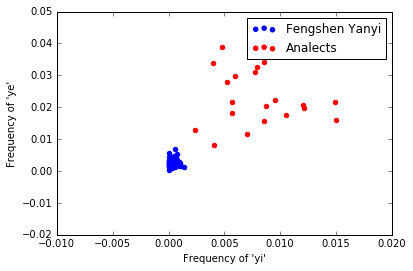You can save changes to your notebook using “File” -> “Save and checkpoint”. Note that Jupyter often saves your changes for you automatically, so if you don’t want to save your changes, you might want to make a copy of your notebook first using “File” -> “Make a Copy”.

You should try to avoid having the same notebook open in two different browser windows or browser tabs at the same time. (If you do this, both pages may try to save changes to the same file, overwriting each other’s work.)

## Exercises¶

Before we start writing programs, we need to get familiar with the Jupyter Notebook programming environment. Check that you can complete the following tasks:

• Run each of the program cells in this notebook that are above this cell on your computer, checking that each of the short programs produces the expected output.
• Clear all of the output using “Cell” -> “All output” -> “Clear”, then run one or two of them again.
• In Jupyter, each cell in a notebook can be run independently. Sometimes the order in which cells are run is important. Try running the following three cells in order, then see what happens when you run them in a different order. Make sure you understand why in some cases you get different results.
In :
```number_of_things = 1
```
In :
```print(number_of_things)
```
```1
```
In :
```number_of_things = number_of_things + 1
print(number_of_things)
```
```2
```
• Some of the programs in this notebook are very simple. Modify and re-run them to perform the following tasks:

• Print out the squares of the numbers 3 through 20 (instead of 1 through 12)
• Print out the cubes of the numbers 3 through 20 (i.e. 3 x 3 x 3 = 27, 4 x 4 x 4 = 64, etc.)
• Instead of printing passages from the first chapter of the Analects, print passages from the Daodejing, and determine the longest passage in it. The URN for the Daodejing is: ctp:dao-de-jing
• Often when programming you’ll encounter error messages. The following line contains a bug; try running it, and look at the output. Work out which part of the error message is most relevant, and see if you can find an explanation on the web (e.g. on StackOverflow) and fix the mistake.

In :
```print("The answer to life the universe and everything is: " + 42)  # This statement is incorrect and isn't going to work
```
```---------------------------------------------------------------------------
TypeError                                 Traceback (most recent call last)
<ipython-input-9-f35205b23c00> in <module>()
----> 1 print("The answer to life the universe and everything is: " + 42)  # This statement is incorrect and isn't going to work

TypeError: Can't convert 'int' object to str implicitly```
• Sometimes a program will take a long time to run – or even run forever – and you’ll need to stop it. Watch what happens to the circle beside the text “Python 3” at the top-right of the screen when you run the cell below.
• While the cell below is running, try running the cell above. You won’t see any output until the cell below has finished running.
• Run the cell below again. While it’s running, interrupt its execution by clicking “Kernel” -> “Interrupt”.
In :
```import time

for number in range(1,21):
print(number)
time.sleep(1)
```
```1
2
3
4
5
6
```
```---------------------------------------------------------------------------
KeyboardInterrupt                         Traceback (most recent call last)
<ipython-input-10-c01c67722f36> in <module>()
3 for number in range(1,21):
4     print(number)
----> 5     time.sleep(1)

KeyboardInterrupt: ```
• The cell below has been set as a “Markdown” cell, making it a text cell instead of a program (“code”) cell. Work out how to make the cell run as a program.

for number in range(1,11):
print(“1/” + str(number) + ” = ” + str(1/number)) # In many programming languages, the symbol “/” means “divided by”

• Experiment with creating new cells below this one. Make some text cells, type something in them, and run them. Copy and paste some code from above into code cells, and run them too. Try playing around with simple modifications to the code.
• (Optional) You can make your text cells look nicer by including formatting instructions in them. The way of doing this is called “Markdown” – there are many good introductions available online.
• Lastly, save your modified notebook and close your web browser. Shut down the Python server process, then start it again, and reload your modified notebook. Make sure you can also find the saved notebook file in your computer’s file manager (e.g. “Windows Explorer”/”File Explorer” on Windows, or “Finder” on Mac OS X).

• Jupyter Notebook Users Manual, Bryn Mawr College Computer Science – This provides a thorough introduction to Jupyter features. This guide introduces many more features than we will need to use, but is a great reference.

As a followup to Google n-grams and pre-modern Chinese, other features of the Google n-gram viewer may help shed some light on the issues with the pre-1950 data for Chinese.

One useful feature is wildcard search, which allows various open-ended searches, the simplest of these being a search for “*”, which plots the most frequent 1-grams in a corpus – i.e. the most commonly occurring words. For example, if we input a single asterisk as our search query on the English corpus, we get the frequencies of the ten most common English words: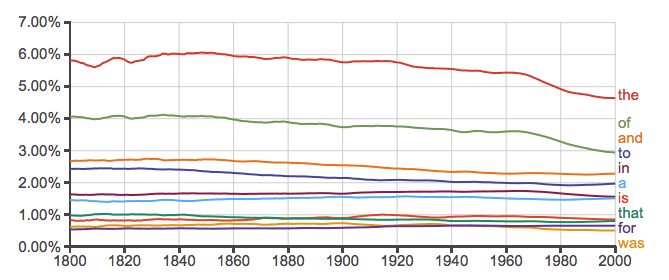The results look plausible at least as far back as 1800, which is what the authors claim to be the reliable part of the data. Earlier than that things get shakier, and before about 1650 things get quite seriously out of hand: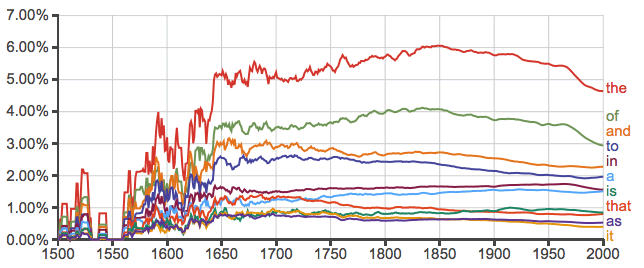Remember, these are the most common terms in the corpus, i.e. the ones for which the data is going to be the most reliable. Now lets look at the equivalent figures for Chinese. Firstly, we can get a nice baseline showing what we would like to see by doing the equivalent search on a relatively reliable part of the data, e.g. 1970 to 2000: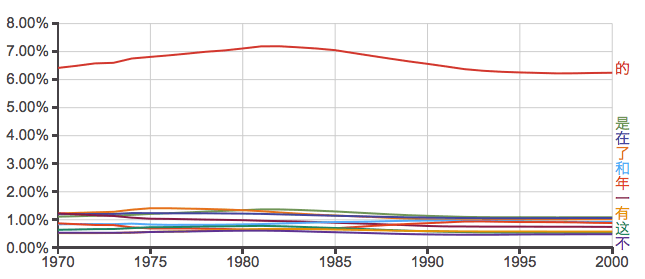This looks good. The top ten 1-grams – i.e. the most frequently occurring terms – are all commonly occurring Chinese words. Now lets try going back to 1800: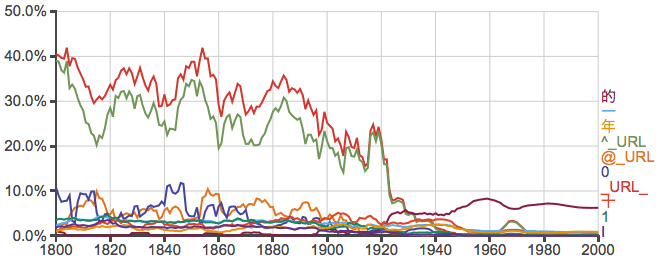Oh dear. From 1800 to 2000, of the ten most frequent 1-grams, more than half are not terms that plausibly occur in pre-modern Chinese texts at all. Note also that the scale of the y axis has now changed: according to this graph, it would appear that up to 40% of terms in pre-1940 texts may have been detected as being URLs or other non-textual content. Unsurprisingly, these problems continue all the way back to 1500: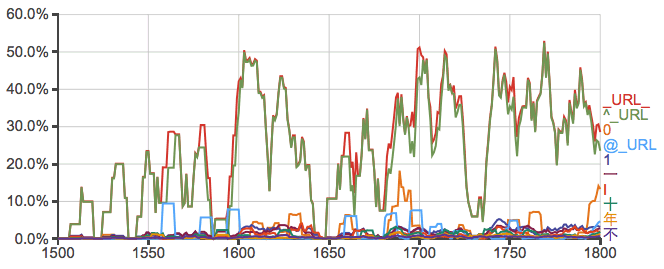It’s unclear what exactly _URL_, ^_URL, and @_URL are supposed to represent as they don’t seem to be documented, and none of them are accepted by the viewer as valid query terms so we can’t easily check what their values are on the English data. Possibly they are just categorization tags that don’t affect the overall counts and thus normalized frequencies, but even so they surely point to serious problems with the data that have caused up to 50% of terms to be so interpreted.

Even aside from these suspect “URLs”, the other most frequent terms returned indicate that three terms not plausibly occurring in pre-modern Chinese texts – “0”, “1”, and “I” – account for anything up to 20% or more of all terms in the pre-1900 data: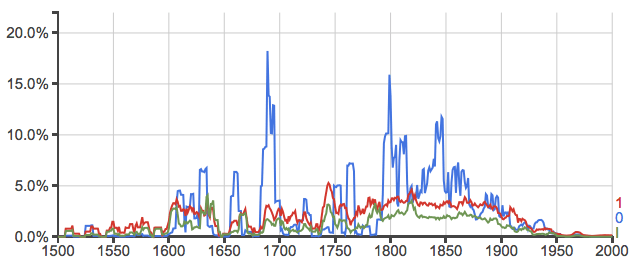Since all the n-gram counts are normalized by the total number of terms, these issues (presumably primarily caused by OCR errors) affect all results for Chinese in any year in which they occur. So it looks as if while 1800 might be a reasonable cut-off for meaningful interpretation of the English data, for the Chinese case 1970 would be a better choice, and any results from before around 1940 will be largely meaningless due to the overwhelming amount of noise.

Update April 18, 2015:

It appears that the @_URL_ and ^_URL_ actually correspond to the terms “@” and “^” (both, presumably, tagged with “URL”), and so these do indeed affect the results: for many years pre-1950, anything up to 60% of all terms in the corpus are the term “^”: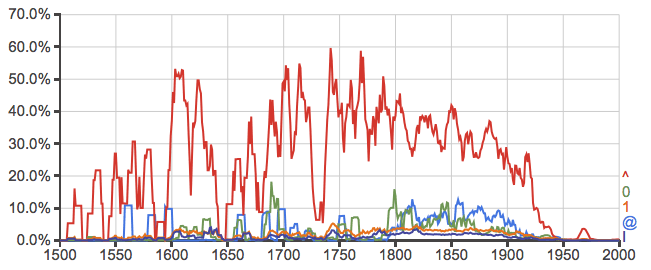It seems that the data used for Chinese fails some fairly basic sanity checks (including “is it in Chinese?”).